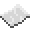# math.log10

math.log10
Function
Syntax
math.log10(
• num : number
)

Returns number
API math
Source Lua (source)

Returns the base-10 logarithms of a number.ExampleGet base-10 logarithms
Get the base-10 logarithms of 5 and print it
Code
```<nowiki>
print(math.log10(5))
</nowiki>
```
Output 0.69897000433602